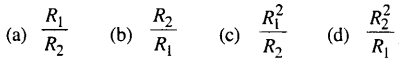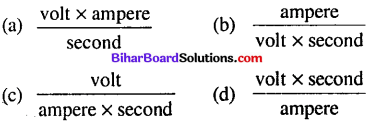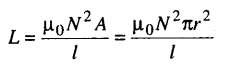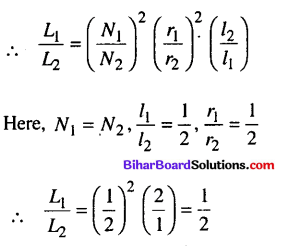# Bihar Board 12th Physics Objective Answers Chapter 6 Electromagnetic Induction

Bihar Board 12th Physics Objective Questions and Answers

## Bihar Board 12th Physics Objective Answers Chapter 6 Electromagnetic Induction

Question 1.
In the figure, galvanometer G gives maximum deflection when(a) magnet is pushed into the coil
(b) magnet is rotated into the coil
(c) magnet is stationary at the centre of the coil
(d) number of turns in the coil is reduced
(a) magnet is pushed into the coilQuestion 2.
The magnetic flux linked with a coil of N turns of area of cross section A held with its plane parallel to the field B is
(a) $$\frac{\mathrm{N} \mathrm{A} B}{2}$$
(b) NAB
(c) $$\frac{\mathrm{N} \mathrm{A} B}{4}$$
(d) zero
(d) zero

Question 3.
Faraday’s laws are consequence of the conservation of
(a) charge
(b) energy
(c) magnetic field
(d) both (b) and (c)
(b) energyQuestion 4.
Two identical coaxial coils P and Q carrying equal amount of current in the same direction are brought nearer. The current in
(a) P increases while in Q decreases
(b) Q increases while in P decreases
(c) both P and Q increase
(d) both P and Q decreases
(d) both P and Q decreases

Question 5.
A closed iron ring is held horizontally and a bar magnet is dropped through the ring with its length along the axis of the ring. The acceleration of the falling magnet is
(a) equalt to g
(b) less than g
(c) more than g
(d) depends on the diameter of the ring and length of magnet
(b) less than gQuestion 6.
Direction of current induced in a wire moving in a magnetic field is found using
(a) Fleming’s left hand rule
(b) Fleming’s right hand rule
(c) Ampere’s rule
(d) Right hand clasp rule
(b) Fleming’s right hand rule

Question 7.
Lenz’s law is a consequence of the law of conservation of
(a) charge
(b) energy
(c) induced emf
(d) induced current
(b) energyQuestion 8.
The direction of induced current in the right loop in the situation shown by the given figure is
(a) along the common axis
(b) along xzy
(c) along xyz
(d) none of these
(c) along xyz

Question 9.
A solenoid is connected to a battery so that a steady current flows through it. If an iron core is inserted into the solenoid, the current will
(a) increase
(b) decrease
(c) remains same
(d) first increase then decrease
(b) decreaseQuestion 10.
A conducting loop is placed in a uniform magnetic field with its plane perpendicular to the field. An emf is induced in the loop if
(a) it is rotated about its axis.
(b) it is rotated about a diameter.
(c) it is not moved.
(d) it is given translational motion in the field.
(b) it is rotated about a diameter.

Question 11.
When a wire loop is rotated in a magnetic field, the direction of induced emf changes in every
(a) one revolution
(b) 1/2 revolution
(c) 1/2 revolution
(d) 2 revolution
(b) 1/2 revolution

Question 12.
A metal plate can be heated by
(a) passing either a direct or alternating current thought the plate.
(b) placing in a time varying magnetic field.
(c) placing in a space varying magnetic field, but does not vary with time.
(d) both (a) and (b) are correct.
(d) both (a) and (b) are correct.

Question 13.
Which of the following does not use the application of eddy current ?
(a) Electric power meters
(b) Induction furnace
(c) LED lights
(d) Magnetic brakes in trains
(c) LED lightsQuestion 14.
Induction furnace make use of
(a) self induction
(b) mutual induction
(c) eddy current
(d) none of these
(c) eddy current

Question 15.
The mutual inductance M12 of a coil I with respect to coil 2
(a) increases when they are brought nearer.
(b) depends on the current passing through the coils.
(c) increases when one of then is rotated about an axis.
(d) both (a) and (b) are correct.
(a) increases when they are brought nearer.

Question 16.
The coefficient of mutual inductance of two coils . depends on
(a) medium between the coils
(b) distance between the two coils
(c) orientation of the two coils
(d) all of these
(d) all of these

Question 17.
Mutual inductance of two coils can be increased by
(a) decreasing the number of turns in the coils
(b) increasing the number of turns in the coils.
(c) winding the coils on wooden cores
(d) none of these
(b) increasing the number of turns in the coils.

Question 18.
If number of turns in primary and secondary coils is increased to two times each, the mutual inductance
(a) becomes 4 times
(b) becomes 2 times
(c) becomes 1/4 times
(d) remain unchanged
(a) becomes 4 timesQuestion 19.
Two conducting circular loops of radii Rx and R2 are placed in the same plane with their centres coinciding. If R1 > R2 the mutual inductance M between them will be directly proportional to(d) $$\frac{R_{2}^{2}}{R_{1}}$$

Question 20.
The physical quantity which is measured in the unit of Wb A”1 is
(a) self inductance
(b) mutual inductance
(c) magnetic flux .
(d) both (a) and (b)
(d) both (a) and (b)

Question 21.
In a coil current falls from 5 A to 0 A in 0.2 s. If an average emf of 150 V is induced, then the self inductance of the coil is
(a) 4H
(b) 2H
(c) 3H
(d) 6H
(d) 6H
Solution:Question 22.
The self inductance of a long solenoid cannot be increased by
(a) increasing its area of cross section
(b) increasing its length
(c) increasing the current through it
(d) increasing the number of turns in it
(c) increasing the current through it

Question 23.
When the rate of change of current is unity, the induced emf is euqal to
(a) thickness of coil
(b) number of turns in coil
(c) coefficient of self inductance
(d) total flux linked with coil
(c) coefficient of self inductance

Question 24.
The self inductance of an inductor coil having 100 turns is 20 mH. The magnetic flux through the cross-section of the coil corresponding to a current of 4 mA is
(a) 2 x 10-5 Wb
(b) 4 x 10-7 Wb
(c) 8 x 10-7 Wb
(d) 8 x 10-5 Wb
(c) 8 x 10-7 Wb

Question 25.
The equivalent quantity of mass in electricity is
(a) current
(b) self inductance
(c) potential
(d) charge
(b) self inductance

Question 26.
If the self inductance of 500 turns coil is 125 mH, then the self inductance of the similar coil of 800 turns is
(a) 48.8 mH
(b) 200 mH
(c) 290 mH
(d) 320 mH
(d) 320 mH
Solution:
(d) As $$\frac{L_{1}}{L_{2}}=\frac{N_{1}^{2}}{N_{2}^{2}}$$
Here self inductance of 500 turns coil = 125 mH
∴ L for the coil of 800 turns
= $$\frac{125}{-500)^{2}}$$ x (800)2 = 320mHQuestion 27.
The unit of inductance is equivalent to(d) $$\frac{\text { volt } \times \text { second }}{\text { ampere }}$$

Question 28.
If the number of turns per unit length of a coil of solenoid is doubled, the self-inductance of the solenoid will
(a) remain unchanged
(b) be halved
(c) be doubled
(d) become four times
(d) become four times

Question 29.
Two solenoids of equal number of turns have their lengths and the radii in the same ratio 1:2. The ratio of their self inductances will be
(a) 1 : 2
(b) 2 : 1
(c) 1 : 1
(d) 1 : 4
(a) 1 : 2
Solution:
(a) Self inductance of a solenoid,where 1 is the length of the solenoid, N is the total number of turns of the solenoid and A is the area of cross-section of the solenoid.Question 30.
The equivalent inductance between A and B is(a) 1H
(b) 4H
(c) 0.8 H
(d) 16 H
(a) 1HQuestion 31.
Two inductors of inductance L each are connected in series with opposite magnetic fluxes. The resultant inductance is (ingore mutual inductance)
(a) zero
(b) L
(c) 2L
(d) 3L
(c) 2L

Question 32.
The energy stored in an inductor of self inductance L henry carrying a current of I ampere is
(a) \frac{1}{2}L2I
(b) \frac{1}{2}LI2
(c) LI2
(d) L2I
(b) \frac{1}{2}LI2Question 33.
A 100 mH coil carries a current of 1 A. Energy stored in its magnetic field is
(a) 0.5 J
(b) 0.05 J
(c) 1 J
(d) 0.1 J
(b) 0.05 J

Question 34.
The working of a generator is based upon
(a) magnetic effect of current
(b) heating effect of current
(c) chemical effect of current
(d) electromagnetic induction
(d) electromagnetic induction

Question 35.
The self inductance L of a solenoid of length / and areas of cross-section A, with a fixed number of turns N increases as
(a) l and A increase.
(b) l decreases and A increases.
(c) l increases and A decreases.
(d) both l and A decrease.